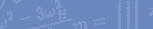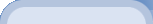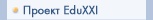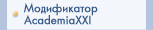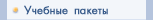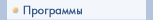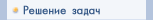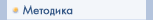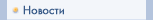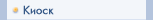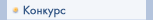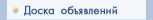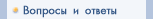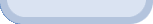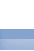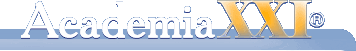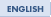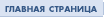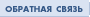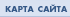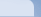# THEORETICAL MECHANICS

25 мая 2003 | Рубрика: Учебные пакеты

 M.N. KIRSANOV TНE SOLVER THEORETICAL MECHANICS PHYSICS AND MATHEMATICS PUBLISHERS International Academic Publishing Company “Nauka” Russian Academy of Sciences, Moscow, 2002 TНE SOLVER “Theoretical Mechanics” is the part of tutorial complexes TНE SOLVER edited by professor A.I. Kirillov. It is designed for computer-based education and consists of this book and software package TНE SOLVER TM.   This book contains 4 parts : STATICS, KINEMATICS and DYNAMICS and and SOLUTIONS in MAPLE V and 17 chapters: Concurrent Force System in a Plane General Case of Forces in a Plane Equilibrium with Friction Forces System in Space Centre of Gravity Kinematics of a Particle Rotational Motion of a Rigid Body Plane Motion of a Rigid Body Resultant Motion of a Particle Motion of a Rigid Body Having One Fixed Point Particle Dynamics Dynamics of a System Analytical Dynamics Vibration of a System Solutions in Maple V (Statics) Solutions in Maple V (Kinematics) Solutions in Maple V (Dynamics) The chapter divided into sections. Each section contains one basic problem with general formulation of the problem, a solution plan, a solution example and ten problems of the same kind. In the most cases the answers of the problem have not only final result but a intermediate results too. It is simplify to find possible error in the solution. TНE SOLVER “Theoretical Mechanics» contains new methodical results of the author and new problems and solutions. Using TНE SOLVER, the student can deeply understand how to solve the basic problems of Theoretical Mechanics because this book concentrate attention on the new and essential. Tutorial complex is useful for tutors. One part of the software is the Problem-Generator, designed by author. Problem-Generator can produce variants of problems with text, figure and answers for test and control in LaTeX. TНE SOLVER «Theoretical Mechanics» can be used at colleges, technical universities, an for distant education. It enables the student to study independently.Copyright: А.И.Кириллов © 2023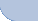Сделано на "Интернет Фабрике"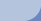Проект EduXXI | Модификатор AcademiaXXI | Учебные пакеты | Программы | Решение задач | Методика | Новости | Киоск | Конкурс | Вопросы и ответы | Доска объявлений Главная страница | Карта сайта | Обратная связь